# Low Cost Palm Vein Authentication System

Authenticate with the veins in your palm.

IntermediateFull instructions provided15 hours7,222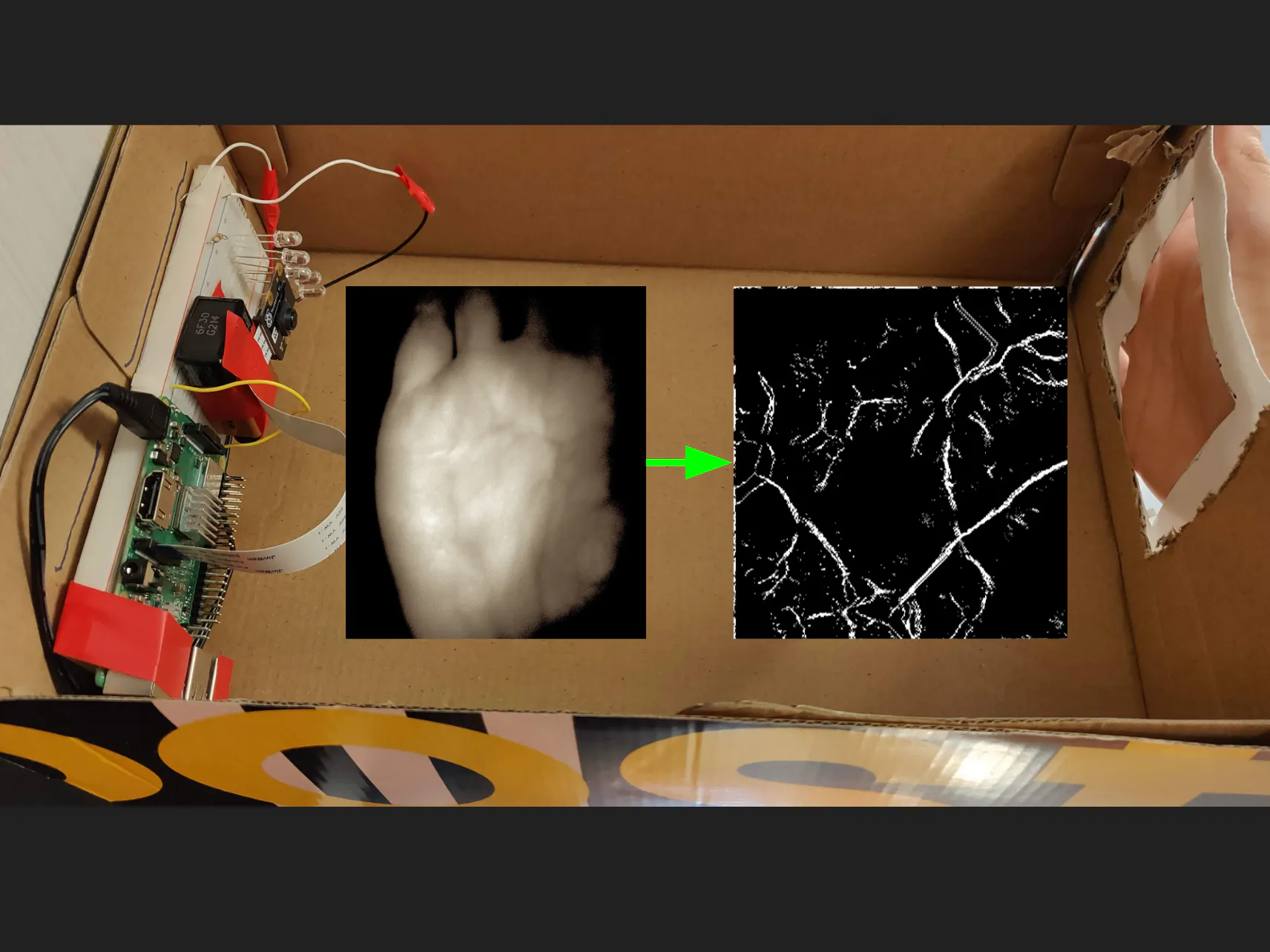## Things used in this project

### Hardware componentsRaspberry Pi 3 Model B
×1×1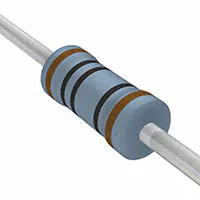Resistor 100 ohm
×1
 Infrared LEDs x50 (950nm)
×1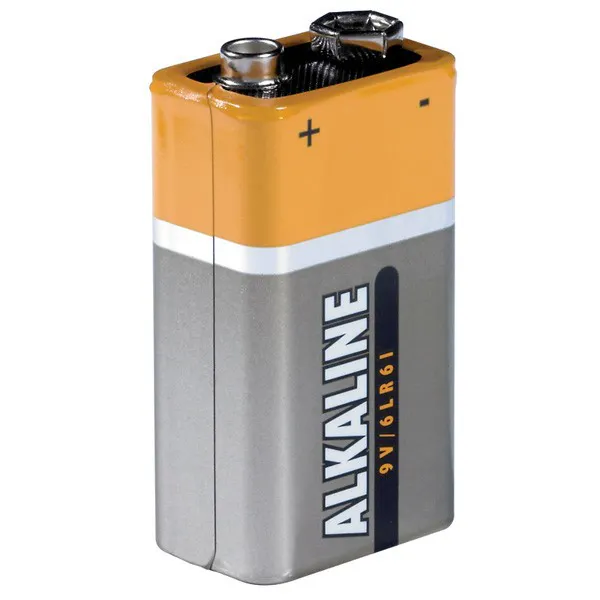9V battery (generic)
×1Jumper wires (generic)
×1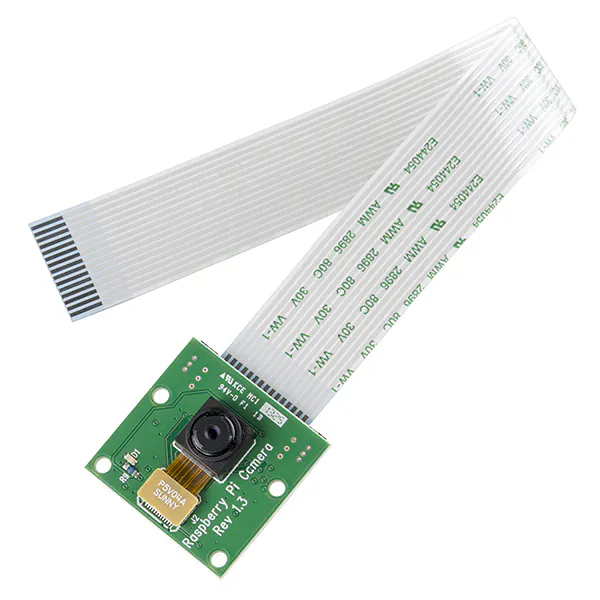Raspberry Pi Camera Module Need the NoIR model - https://www.raspberrypi.org/products/pi-noir-camera-v2/
×1
 Shoe Box
×1

### Software apps and online servicesOpenCV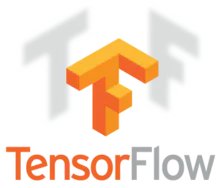TensorFlow

## Code

### palm.py

Python
```import cv2
import numpy as np
import tensorflow as tf
from tensorflow import keras
from PIL import Image

print "training model"

classes = ["left", "right"]

num_right_train = 20
num_left_train = 20

pic = np.array(Image.open("train/right_thr" + str(0) + ".jpg"))
train_images = np.array([pic])
train_labels = np.array(*num_right_train + *num_left_train)

for i in range(1, num_right_train):
pic = np.array(Image.open("train/right_thr" + str(i) + ".jpg"))
train_images = np.vstack((train_images, np.array([pic])))

for i in range(num_left_train):
pic = np.array(Image.open("train/left_thr" + str(i) + ".jpg"))
train_images = np.vstack((train_images, np.array([pic])))

train_images = train_images / 255.0

model = keras.Sequential([
keras.layers.Flatten(input_shape=(600, 600)),
keras.layers.Dense(64, activation=tf.nn.relu),
keras.layers.Dense(2, activation=tf.nn.softmax)
])

loss='sparse_categorical_crossentropy',
metrics=['accuracy'])

model.fit(train_images, train_labels, epochs=5)

while raw_input("took pic? [y/n] ") == "y":
# noise
gray = cv2.cvtColor(img, cv2.COLOR_BGR2GRAY)
noise = cv2.fastNlMeansDenoising(gray)
noise = cv2.cvtColor(noise, cv2.COLOR_GRAY2BGR)
print "reduced noise"

# equalist hist
kernel = np.ones((7,7),np.uint8)
img = cv2.morphologyEx(noise, cv2.MORPH_OPEN, kernel)
img_yuv = cv2.cvtColor(img, cv2.COLOR_BGR2YUV)
img_yuv[:,:,0] = cv2.equalizeHist(img_yuv[:,:,0])
img_output = cv2.cvtColor(img_yuv, cv2.COLOR_YUV2BGR)
print "equalized hist"

# invert
inv = cv2.bitwise_not(img_output)
print "inverted"

# erode
gray = cv2.cvtColor(inv, cv2.COLOR_BGR2GRAY)
erosion = cv2.erode(gray,kernel,iterations = 1)
print "eroded"

# skel
img = gray.copy()
skel = img.copy()
skel[:,:] = 0
kernel = cv2.getStructuringElement(cv2.MORPH_CROSS, (5,5))
iterations = 0

while True:
eroded = cv2.morphologyEx(img, cv2.MORPH_ERODE, kernel)
temp = cv2.morphologyEx(eroded, cv2.MORPH_DILATE, kernel)
temp  = cv2.subtract(img, temp)
skel = cv2.bitwise_or(skel, temp)
img[:,:] = eroded[:,:]
if cv2.countNonZero(img) == 0:
break

print "skeletonized"
ret, thr = cv2.threshold(skel, 5,255, cv2.THRESH_BINARY);

cv2.imwrite("thr.jpg", thr)

# predict
pic = np.array(Image.open("thr.jpg"))
test_images = np.array([pic])
print "predicting result"
predictions = model.predict(test_images)
print predictions